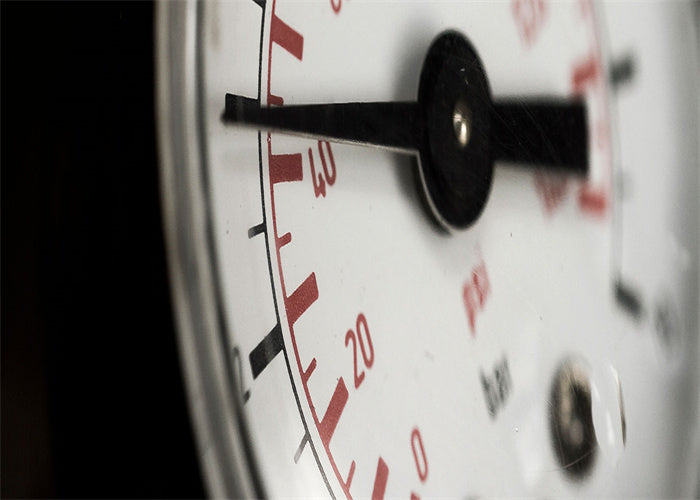# What Is the Difference Between Absolute Pressure, Gauge Pressure and Differential Pressure?

·In addition to temperature, the pressure is one of the most important physical state variables. The pressure is defined as the force (F N) acting uniformly on the limited area (A). Different types of pressure are distinguished only by reference pressure.

Absolute Pressure

The clearest reference pressure is zero pressure, which exists in the airless space of the universe. The pressure associated with this reference pressure is called absolute pressure. For the required differentiation from other types of pressure, it is denoted with the index “abs”, which is derived from the Latin “absolutus”, meaning detached, independent.

Atmospheric Pressure

The probably most important pressure for life on earth is the atmospheric pressure, pamb (amb = ambiens = ambient). It is produced by the weight of the atmosphere surrounding the earth, the height of the atmosphere surrounding the earth is approx. 500 kilometers. Up to this altitude, at which the absolute pressure pabs = zero, its magnitude continuously decreases. In addition, atmospheric pressure is affected by weather changes, which can be known from the daily weather report. At sea level, pamb averages 1,013.25 hectopascals (hpa), which is equivalent to 1,013.25 millibars (mbar). With “cyclones” and “anticyclones”, this pressure varies by about 5 %.

Differential Pressure

The difference between two pressures, p1 and p2, is known as the pressure differential, Δp = p1 – p2. In cases where the difference between two pressures itself represents the measured variable, one refers to the differential pressure, p1,2.

Therefore, in order to measure the differential pressure, at first two different pressures are captured in a measuring instrument. Only if the measured values differ from each other, will a differential pressure be indicated. Instances where it is required to measure differential pressure are, for example, level measurement and monitoring applications.

Overpressure (Gauge Pressure)

The most commonly used measurement pressure in the technical field is the atmospheric pressure difference, Pe (e = excedens = exceed). It is the difference between an absolute pressure, pabs, and the relevant (absolute) atmospheric pressure (pe = pabs – pamb) and is known, in short, as the overpressure or gauge pressure.

When the absolute pressure is greater than atmospheric pressure, it indicates positive overpressure. In the opposite case, people would say negative overpressure.

The formula symbols “abs”, “amb” and “e” clearly define the reference point of each pressure. They are only attached to the formula letter p, not to the unit symbol.Controller